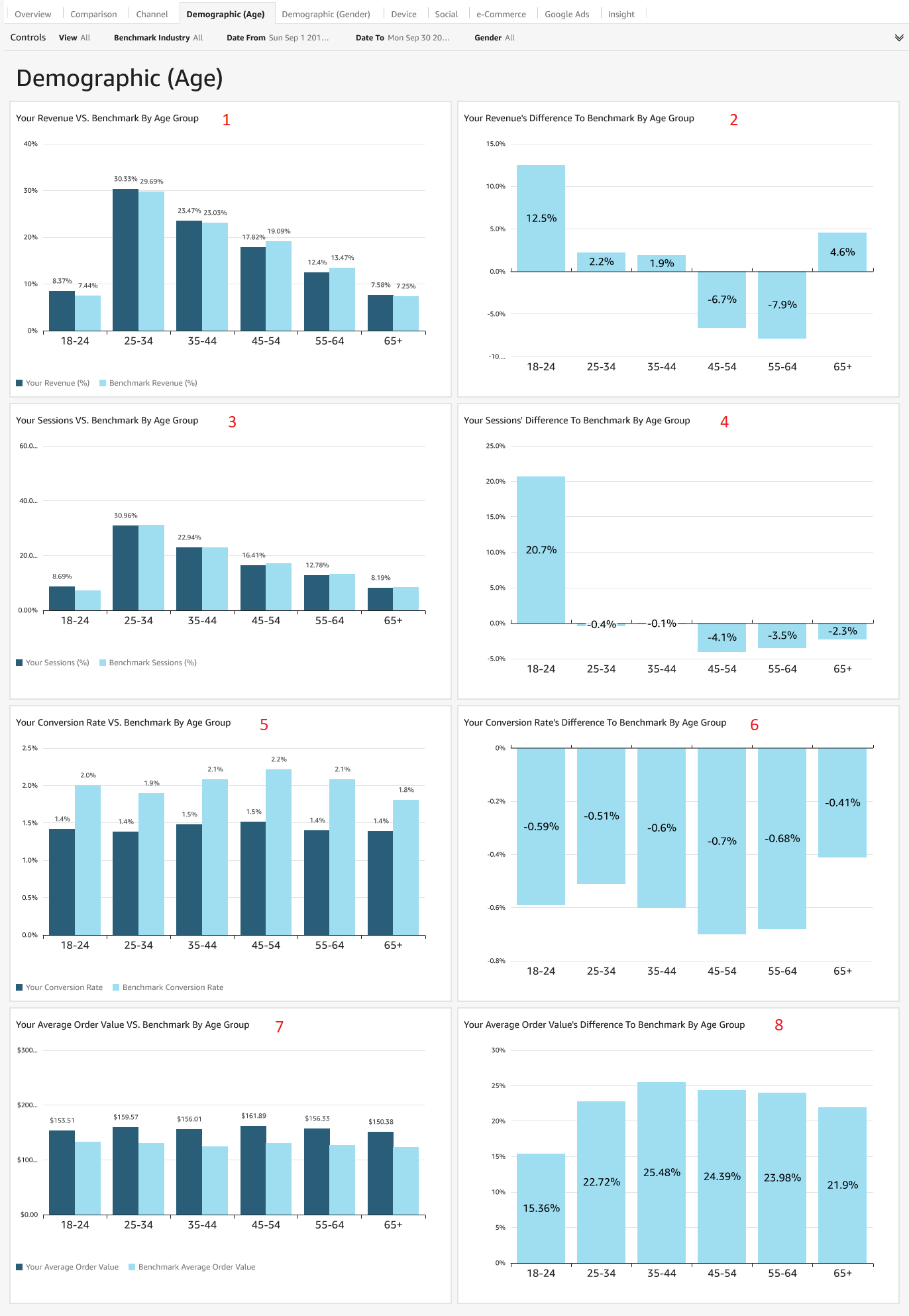This tab shows key metrics across age groups, allowing you an in-depth view on how you perform with audiences of different age groups. The dark blue lines represent your data, and the light blue lines are the benchmark's data. You will also be able to filter by gender to see only females or males, or a combination.1. Your Revenue vs Benchmark by Age Group: This bar chart shows your revenue broken down by age group, showing you which age group makes up the highest revenue. Revenue is calculated as a percent of total, for example the 18-24 age group makes up 8.37% of your total revenue for the selected time period, compared to the benchmark's 7.44%.

2. Your Revenue's Difference to Benchmark by Age Group: This bar chart shows your revenue's difference to the benchmark. As an example, your 18-24 age group's revenue is 8.37%, while the benchmark is 7.44%. The difference is calculated as [(8.37-7.44)/7.44)] x 100 = 12.5%, which is shown as the first bar chart.

3. Your Sessions vs. Benchmark by Age Group: This bar chart shows your sessions broken down by age groups, showing you which age group makes up the highest sessions.

4. Your Sessions Difference to Benchmark by Age Group: This bar chart shows your sessions difference to the benchmark. As an example, your 18-24 age group conversion rate is 8.69%, while the benchmark is 7.2%. The difference is calculated as (8.69 -7.2)/7.2 x 100 = 20.7%, which is shown as the first bar chart.

5. Your Conversion Rate vs Benchmark by Age Group: This bar chart shows your average conversion rate broken down by age group. For the 18-24 age group, your conversion rate is 1.4%, vs the Benchmark's 2.0%.

6. Your Conversion Rate's Difference to Benchmark by Age Group: This bar chart shows your average conversion rate difference to the benchmark. As an example, your conversion rate for 18-24 is 1.4%, while the benchmark is 2.0% The difference is calculated as (1.4-2.0)/2.0 x 100 = -0.59%, which is shown as the first bar chart.

7. Your Average Order Value (AOV) vs Benchmark by Age Group: This bar chart shows your AOV broken down by age groups. In this example, the 18-24 age group has an AOV of \$153.51 compared to the benchmark's AOV of \$133.06.

8. Your Average Order Value's (AOV) Difference to Benchmark by Age Group: This bar chart shows your average order value's difference to the benchmark. As an example, your 18-24 age group's average order value is \$153.51, while the benchmark is \$133.06. The difference is calculated as [(153.51-133.06)/133.06)] x 100 = 15.36%, which is shown as the first bar chart.